# Effective computation of zeta-functions

The Weil conjectures (proved by Dwork, Grothendieck, Deligne et al.) assert that for any algebraic variety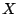over a finite field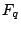, the power series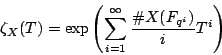is a rational function of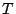; giving explicit methods for computing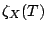is a fundamental problem in computational number theory. In principle, one can do this by simply giving bounds on the degrees of the numerator and denominator, then explicitly computing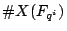for enough values of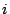. (E.g., ifis projective, one can choose a projective embedding of, defined by certain equations, then count the number of points in projective space satisfying those equations.) In practice, this enumeration gives an algorithm which requires time exponential in the complexity'' of(e.g., the logarithm of the base field size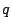, and the degrees of the equations defining) and thus can only be completed for relatively small examples.

Below we describe the current state of knowledge regarding subexponential methods for computing zeta functions. We omit mention of methods which improve on pure exhaustion but are still exponential, such as Mestre's baby step-giant step'' method for computing the zeta function of an elliptic curve.

Back to the main index for L-functions and Random Matrix Theory.